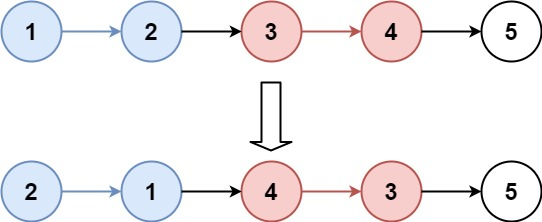# 25. Reverse Nodes in k-Group | LeetCode

## 25. Reverse Nodes in k-Group

Given a linked list, reverse the nodes of a linked list k at a time and return its modified list.

k is a positive integer and is less than or equal to the length of the linked list. If the number of nodes is not a multiple of k then left-out nodes, in the end, should remain as it is.

You may not alter the values in the list's nodes, only nodes themselves may be changed.

Example 1:```Input: head = [1,2,3,4,5], k = 2
Output: [2,1,4,3,5]
```

Example 2:```Input: head = [1,2,3,4,5], k = 3
Output: [3,2,1,4,5]
```

Example 3:

```Input: head = [1,2,3,4,5], k = 1
Output: [1,2,3,4,5]
```

Example 4:

```Input: head = , k = 1
Output: 
```

Constraints:

• The number of nodes in the list is in the range `sz`.
• `1 <= sz <= 5000`
• `0 <= Node.val <= 1000`
• `1 <= k <= sz`

### Solution

/**
* struct ListNode {
*     int val;
*     ListNode *next;
*     ListNode() : val(0), next(nullptr) {}
*     ListNode(int x) : val(x), next(nullptr) {}
*     ListNode(int x, ListNode *next) : val(x), next(next) {}
* };
*/
class Solution
{
public:
{
int cnt = 0;
while (temp != NULL)
{
cnt++;
temp = temp->next;
}
cout << cnt << " ";
if (cnt >= k)
{
return true;
}
return false;
}
{
{
}
ListNode *pre = NULL;
int t = k;
ListNode *temp;
while (curr != NULL && t--)
{
temp = curr->next;
curr->next = pre;
pre = curr;
curr = temp;
}
if (temp != NULL)
{
if (count(temp, k))
{
}
else
{